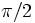# Classification of wallpaper groups

## Statement

This article completely classifies all the space groups in two dimensions, also called the wallpaper groups. The classification up to affine space type is the same as the classification up to crystallographic space type, and there are a total of seventeen types.

The seventeen types are as follows:

IUC name Description of non-translation generators Point group Splits Full automorphism group Orientation-preserving$p1$ None Trivial Yes No Yes$pg$ Glide reflection Two-element subgroup generated by reflection No No No$pm$ Reflection Two-element subgroup generated by reflection Yes No No$cm$ Reflection and glide reflection Klein four-group generated by two reflections No No No$p2$ Rotation by$\pi$ Two-element subgroup generated by$\pi$-rotation Yes Yes Yes$pmg$ Reflection and rotation by$\pi$ Klein four-group generated by two reflections No No No$cmm$ Reflection, rotation by$\pi$ with rotation center off reflection axis Klein four-group generated by two reflections No No No$pmm$ Two orthogonal reflections Klein four-group generated by two reflections Yes Yes No$pgg$ Rotation by$\pi$, glide reflection Klein four-group generated by two reflections No No No$p4$ Rotation by$\pi/2$ Four-element subgroup generated by$\pi/2$-rotation Yes No Yes$p4g$ Rotation by$\pi/2$, Reflection Dihedral group of order eight No No No$p3$ Rotation by$2\pi/3$ Three-element subgroup generated by rotation Yes No Yes$p3m1$ Rotation by$2\pi/3$, Reflection Dihedral group of order six Yes No No$p31m$ Rotation by$2\pi/3$, Reflection, with rotation center off reflection axis Dihedral group of order six No No No$p6$ Rotation by$\pi/3$ Six-element subgroup generated by rotation Yes No$p6m$ Rotation by$\pi/3$, Reflection Dihedral group:D12 Yes Yes No

## Facts used

1. Crystallographic restriction: This is the main lemma used in the classification, and it states that if a lattice possesses a nontrivial rotational symmetry, then it is spanned by two shortest vectors of equal length at an angle of$\pi/2, \pi/3, 2\pi/3$. Note that the case of$\pi/3$ and$2\pi/3$ gives equivalent lattices.